Most Affordable JEE | NEET | 8,9,10 Preparation by Kota's Top IITian Doctor Faculties

# NCERT Solutions for Class 10 Maths chapter 4 - Quadratic Equations

Class 10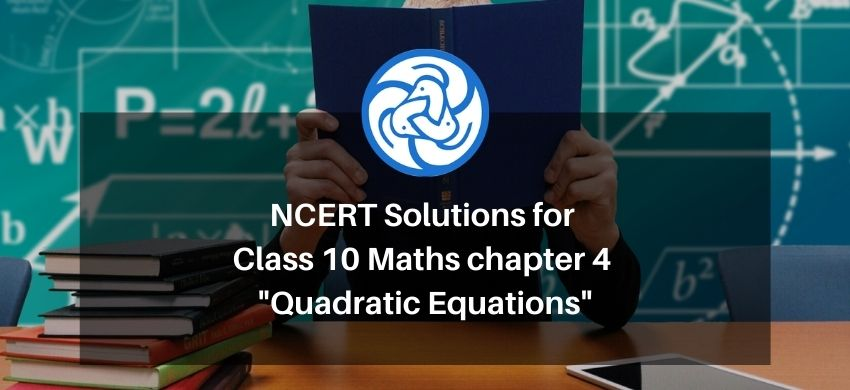`

Hey, are you a class 10 student and looking for ways to download NCERT Solutions for Class 10 Maths chapter 4 "Quadratic Equations"? If yes. Then read this post till the end.

In this article, we have listed NCERT solutions for class 10 Maths Chapter 4 in PDF that is prepared by Kota’s top IITian’s Faculties by keeping Simplicity in mind.

If you want to learn and understand class 10 Maths chapter 4 "Quadratic Equations" in an easy way then you can use these solutions PDF.

NCERT Solutions helps students to Practice important concepts of subjects easily. Class 10 Maths solutions provide detailed explanations of all the NCERT exercise questions that students can use to clear their doubts instantly.

If you want to score high in your class 10 Maths Exam then it is very important for you to have a good knowledge of all the important topics, so to learn and practice those topics you can use eSaral NCERT Solutions.

So, without wasting more time Let’s start.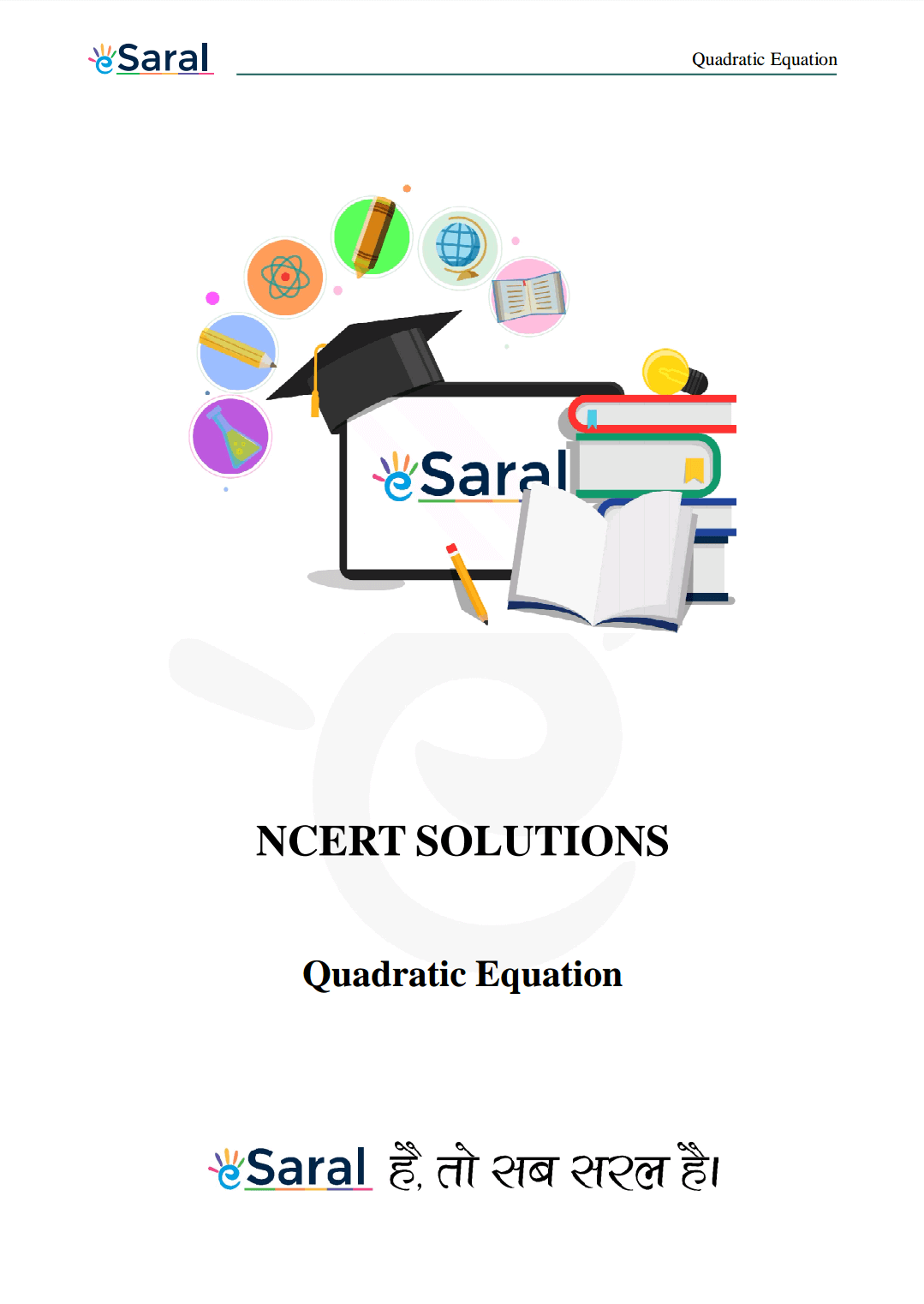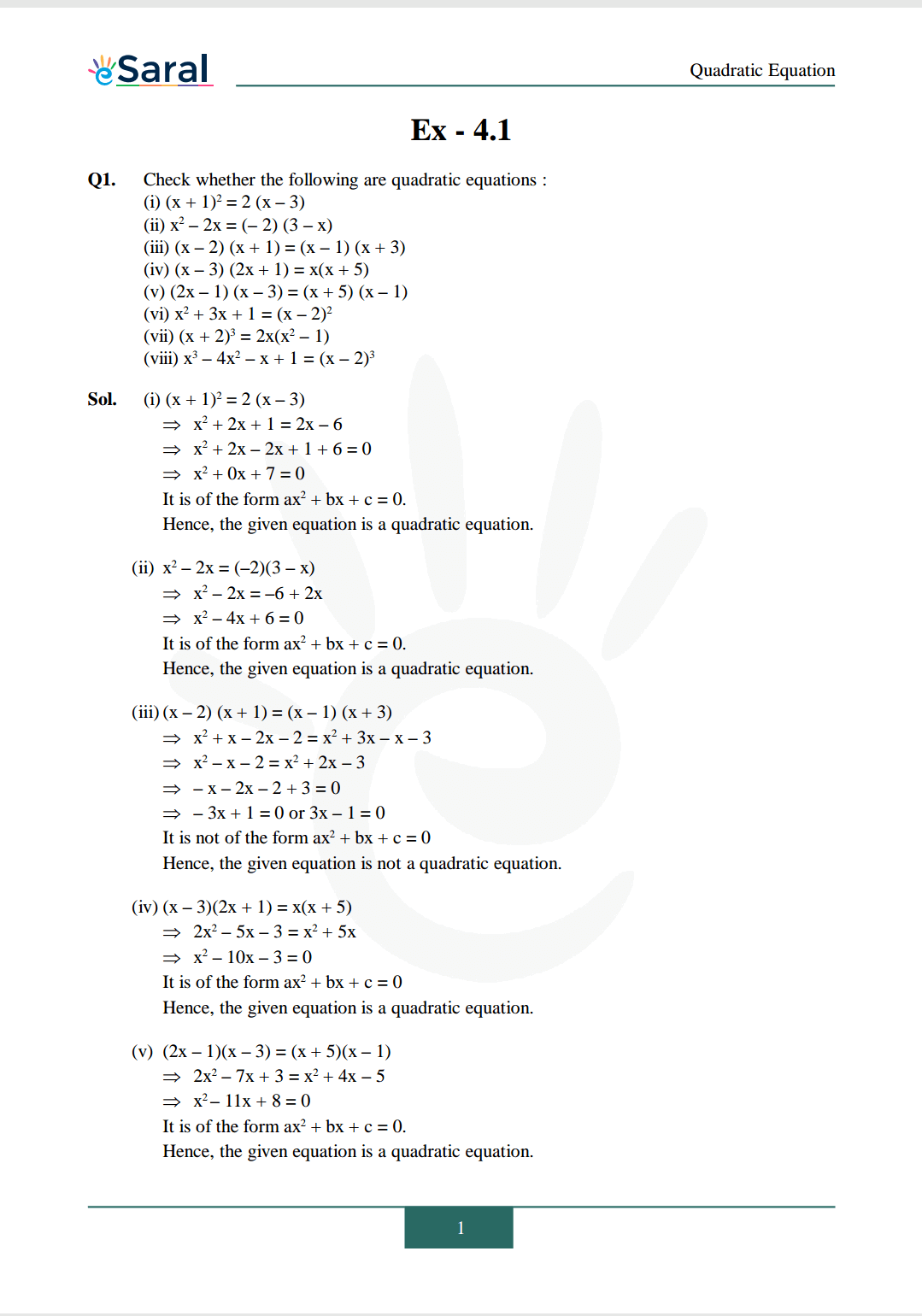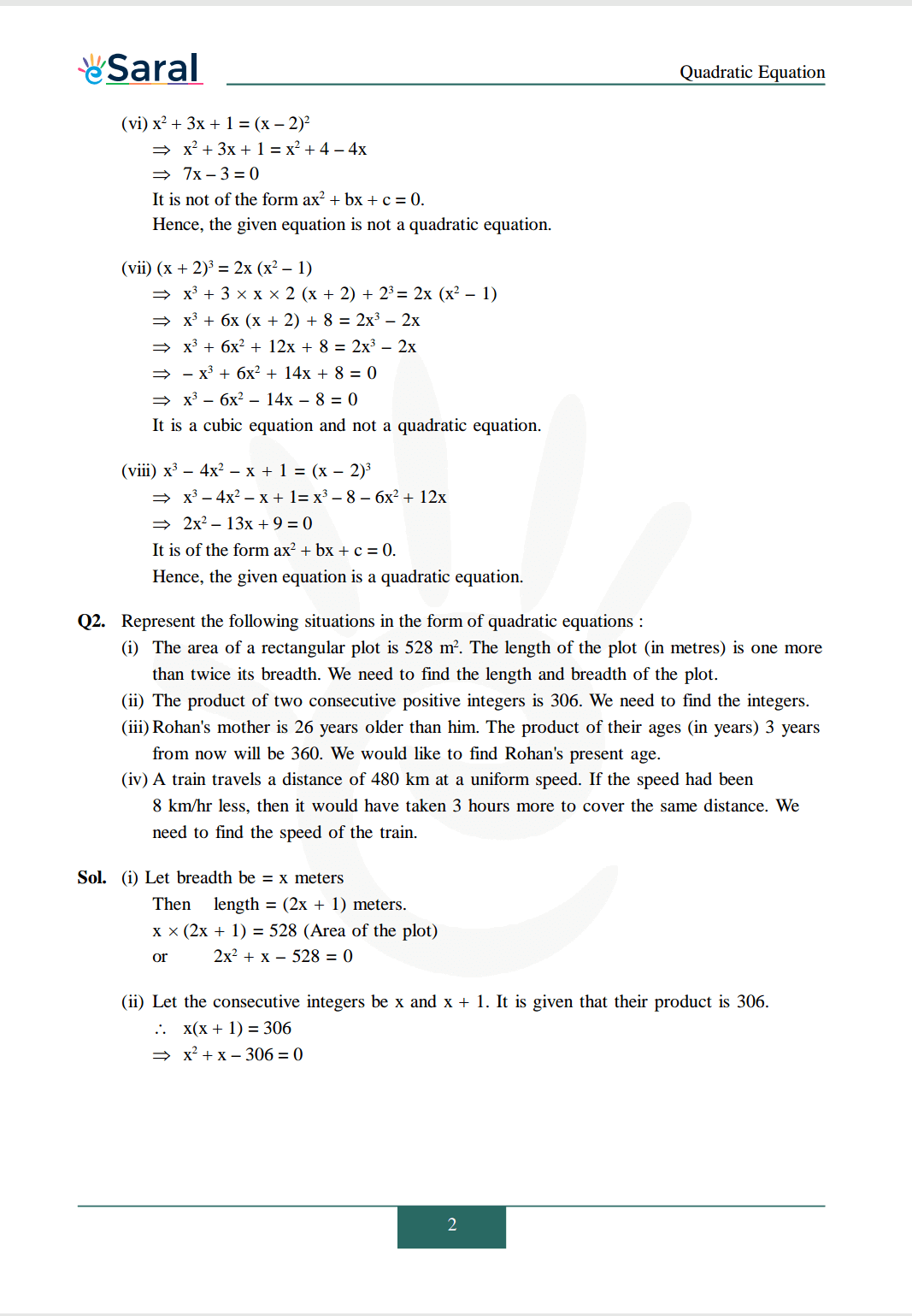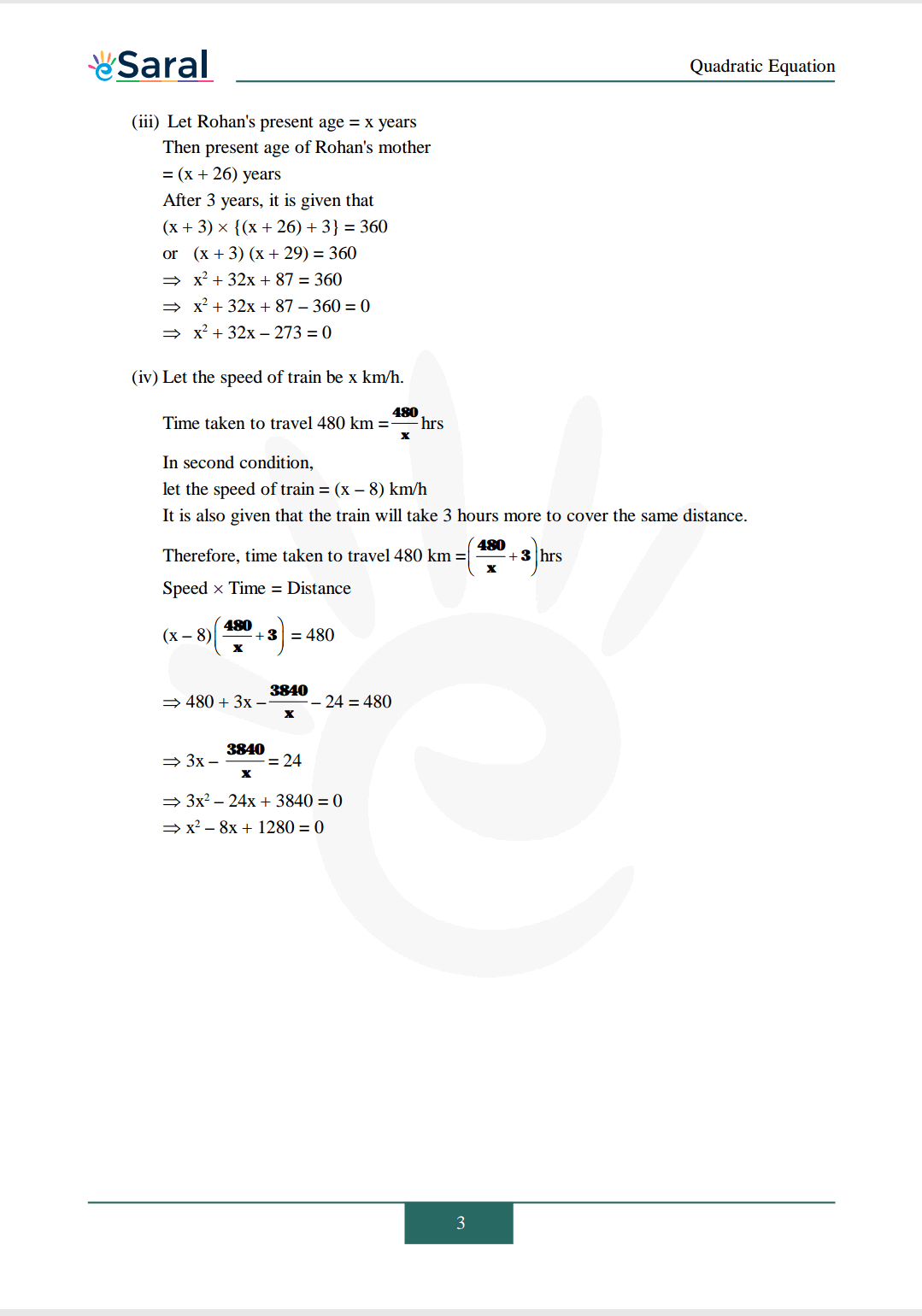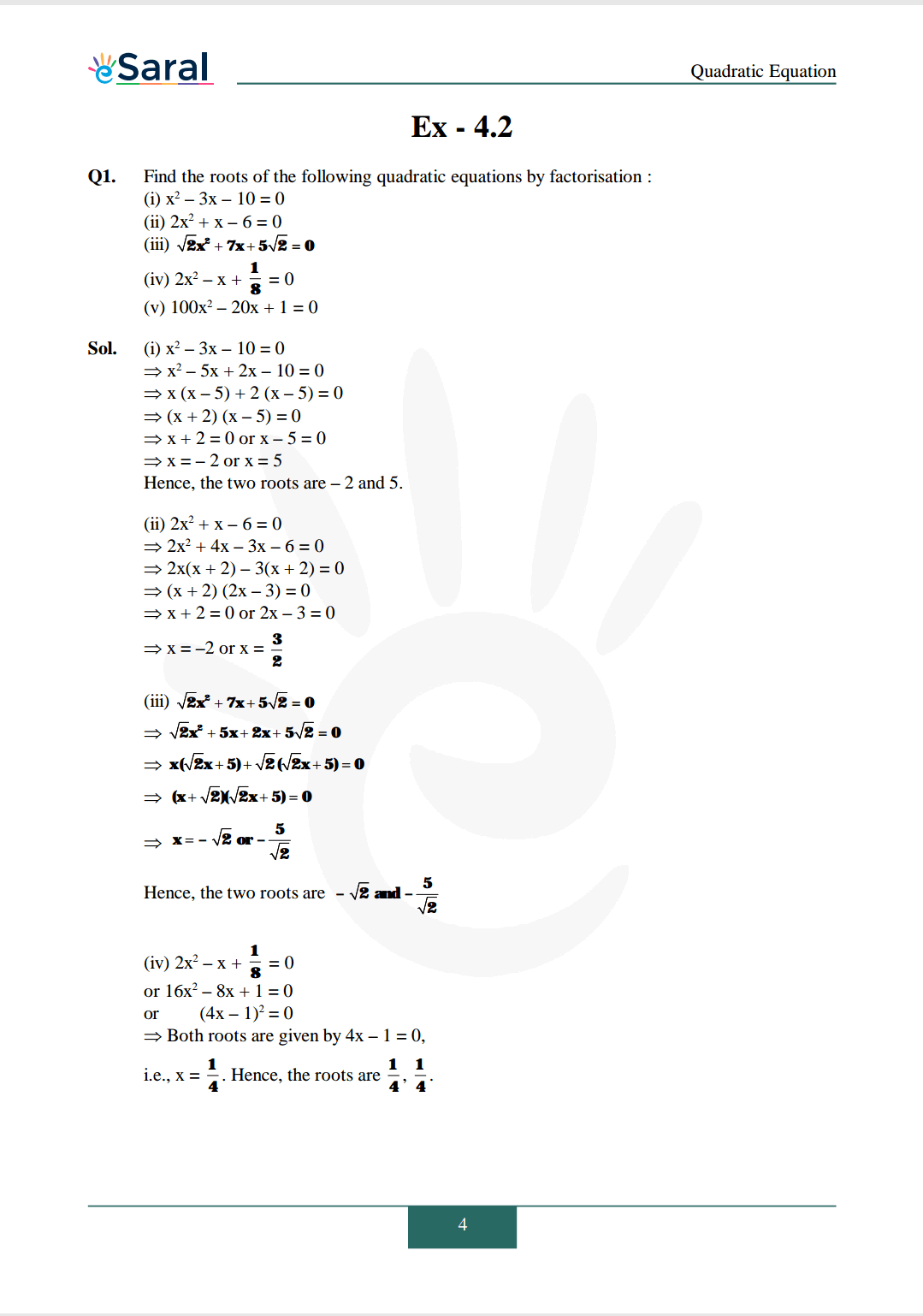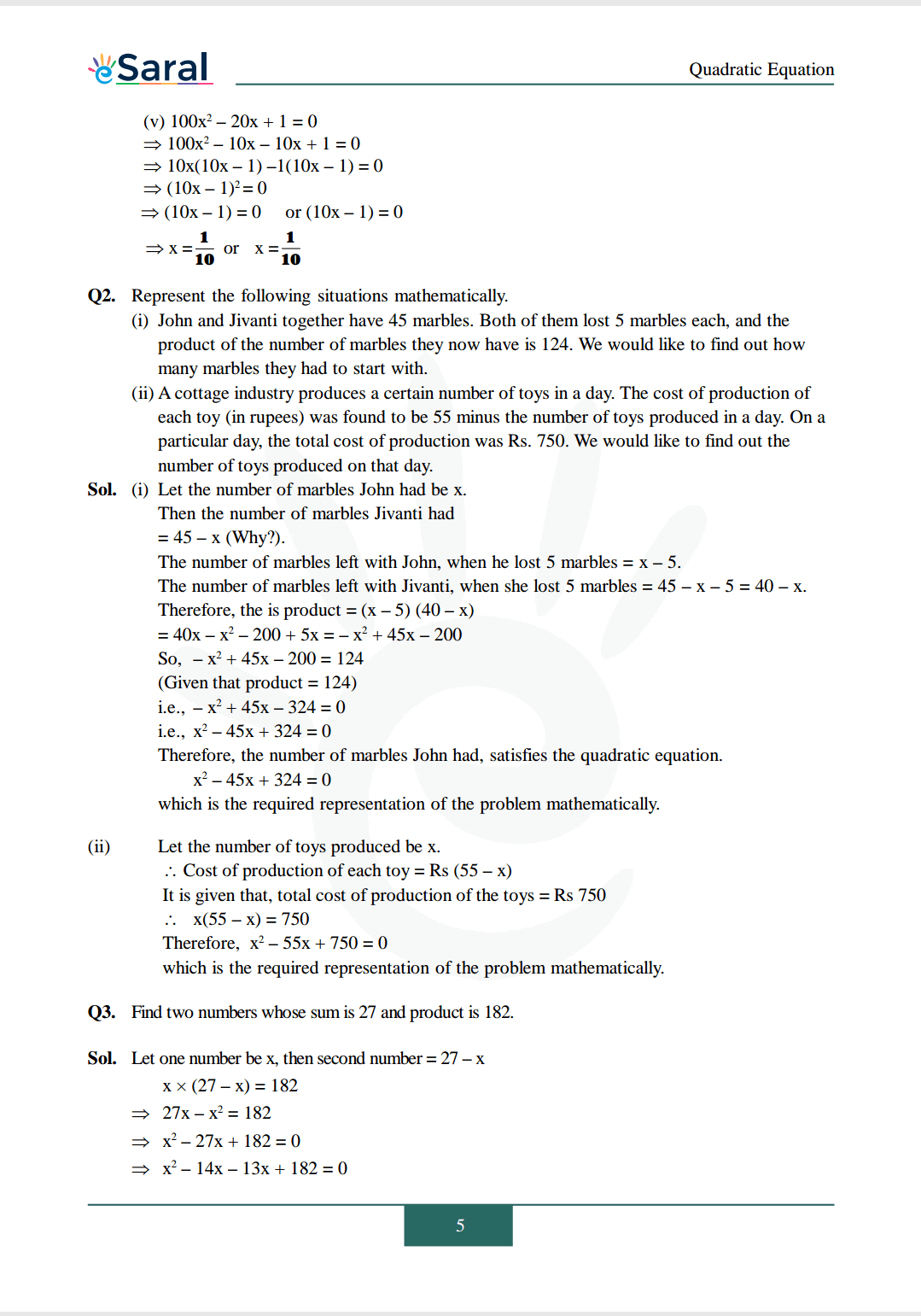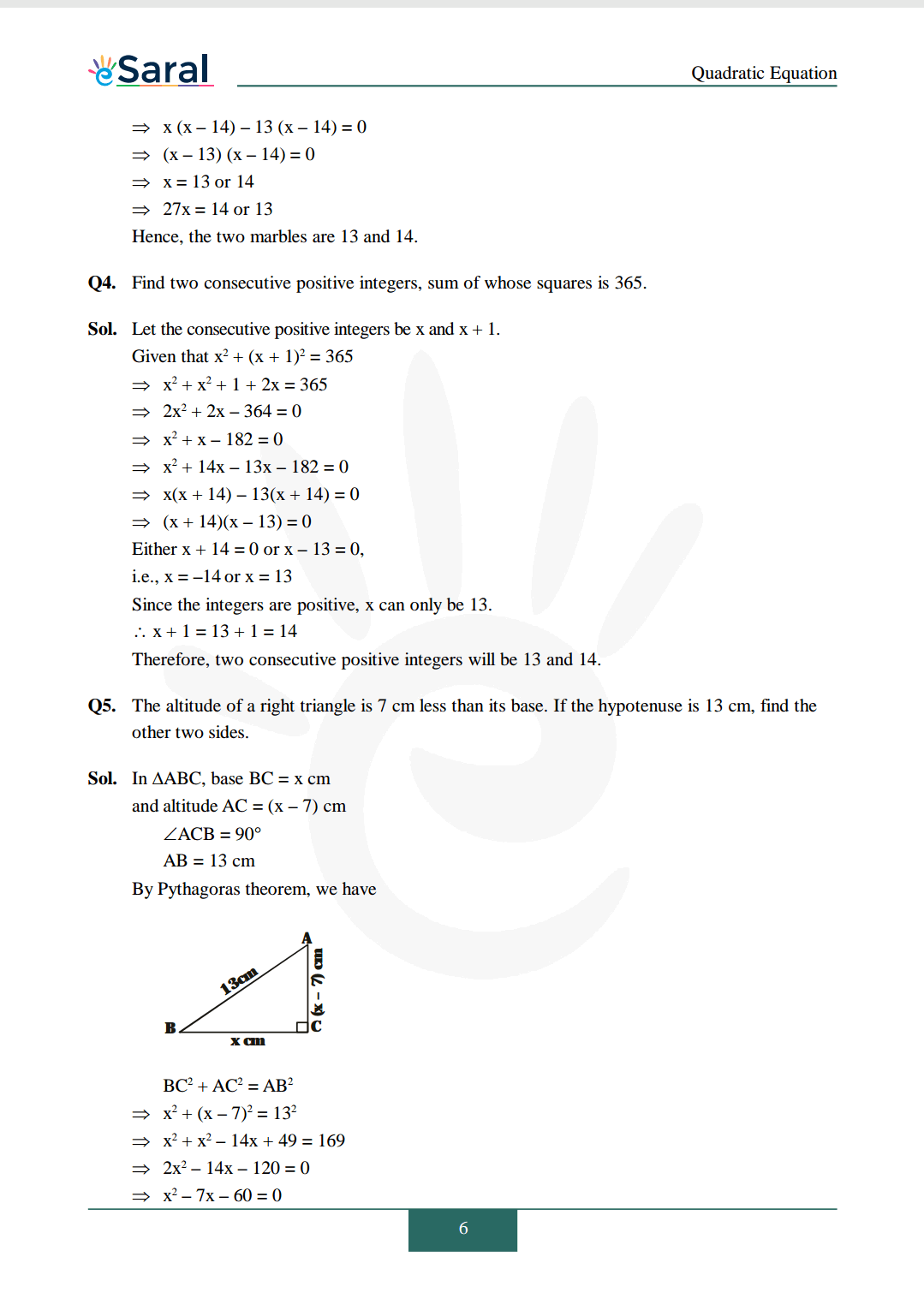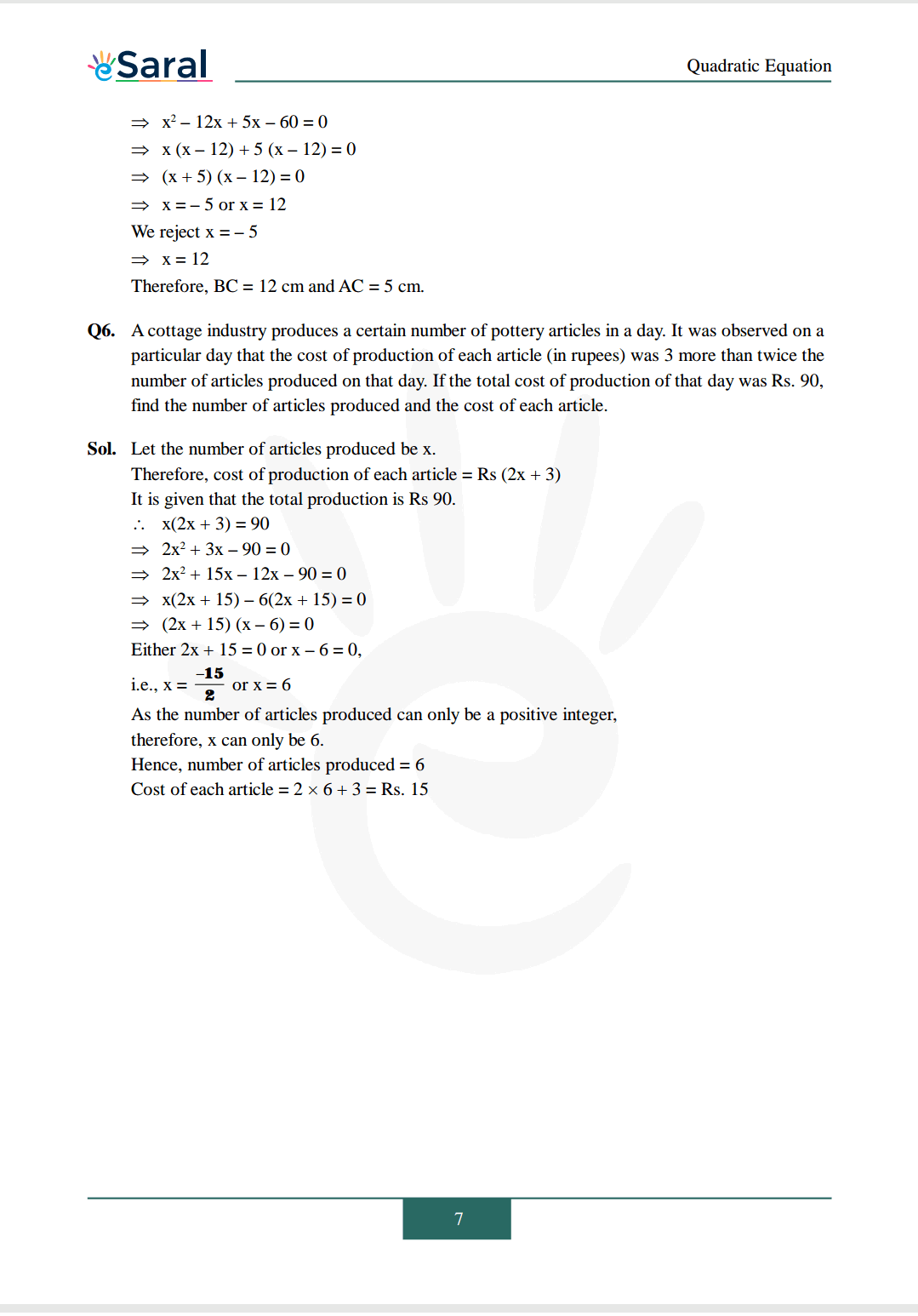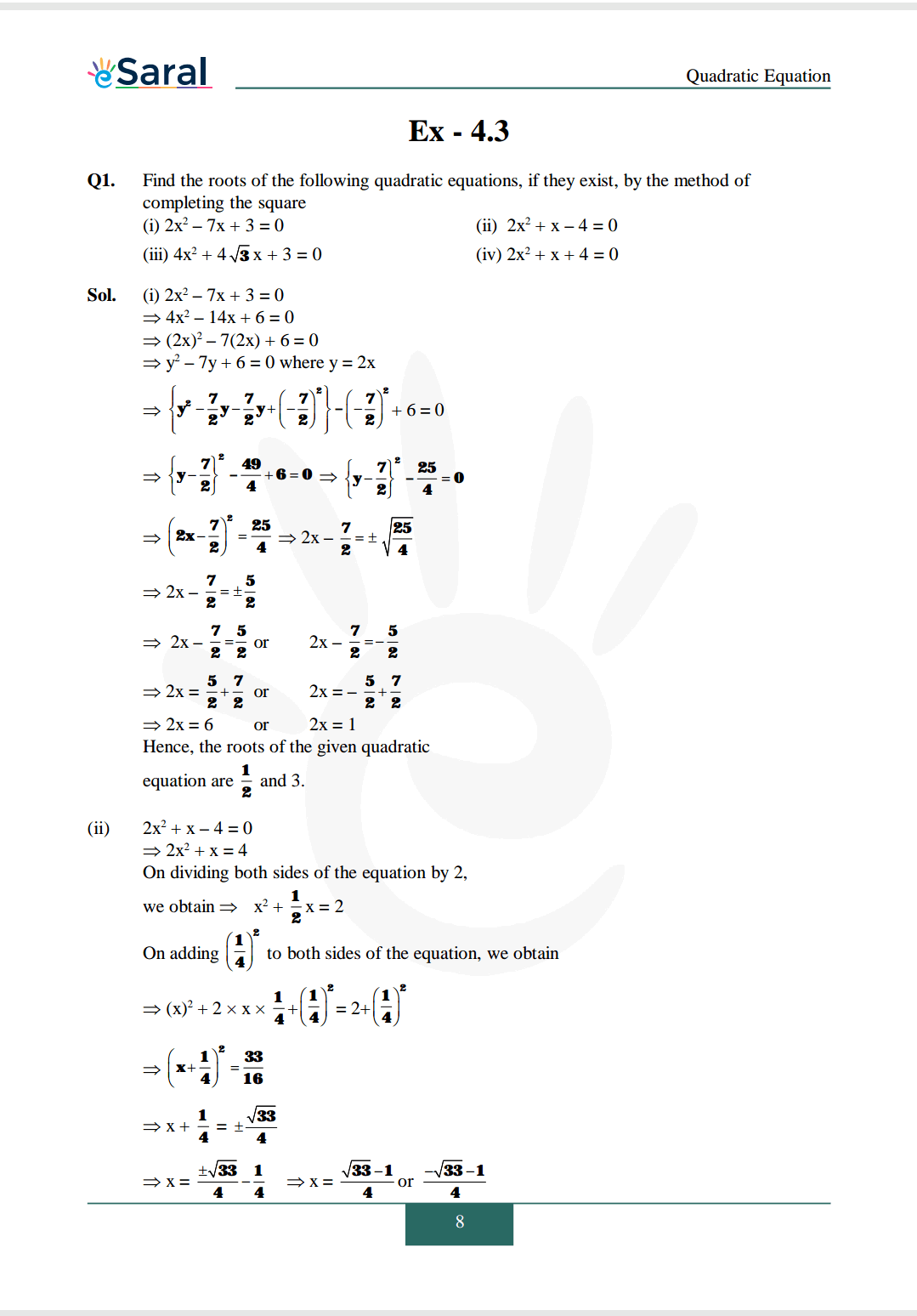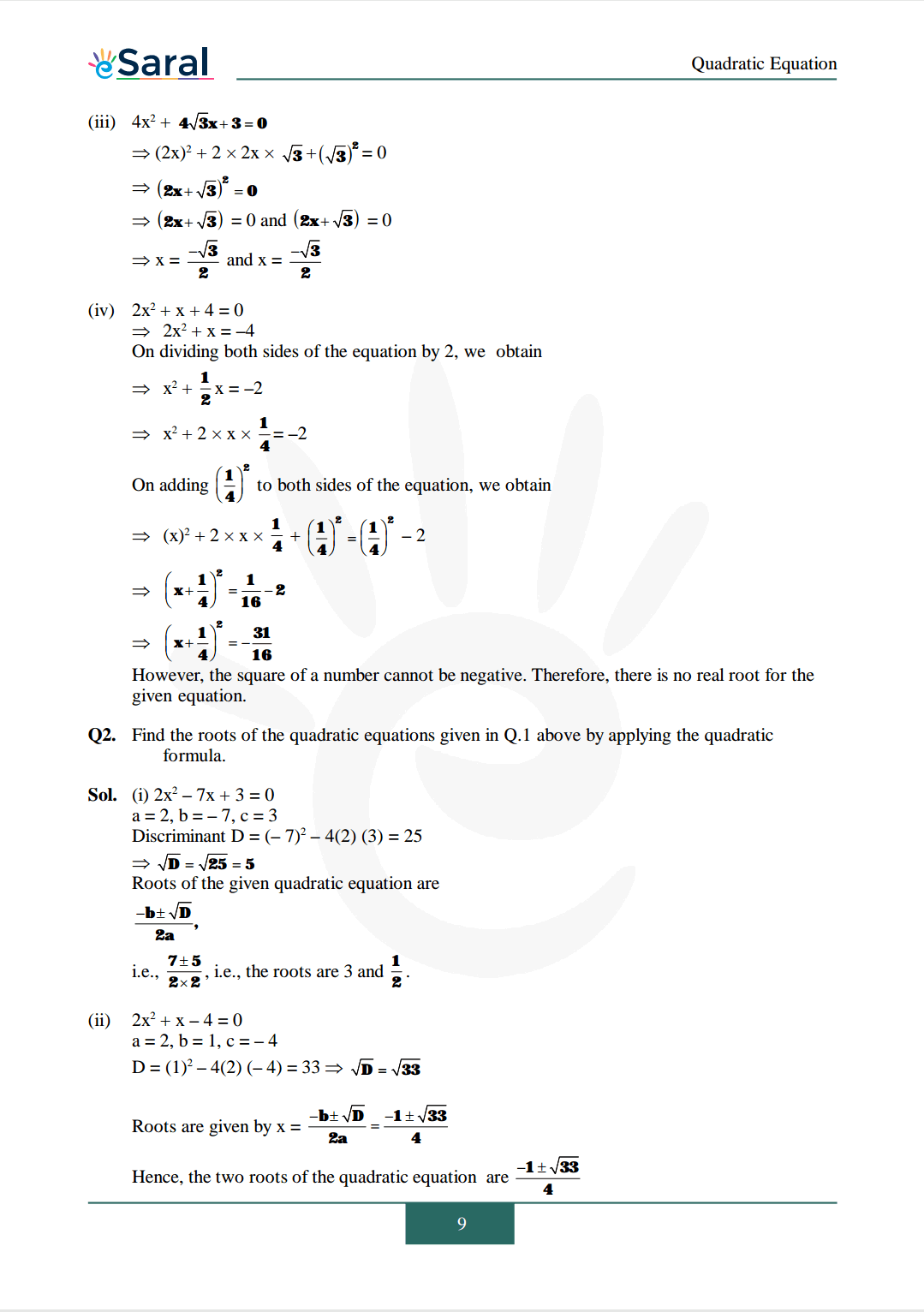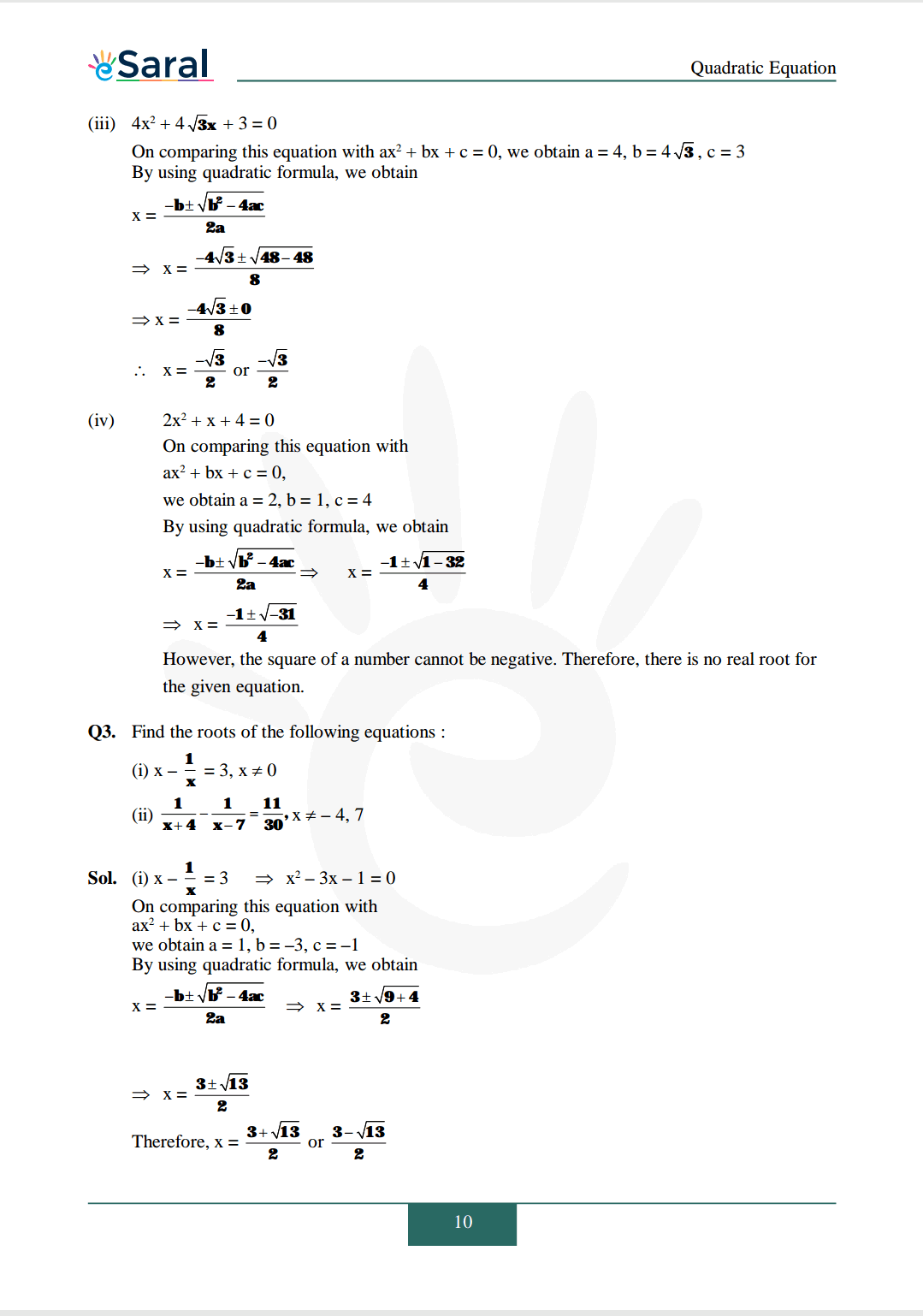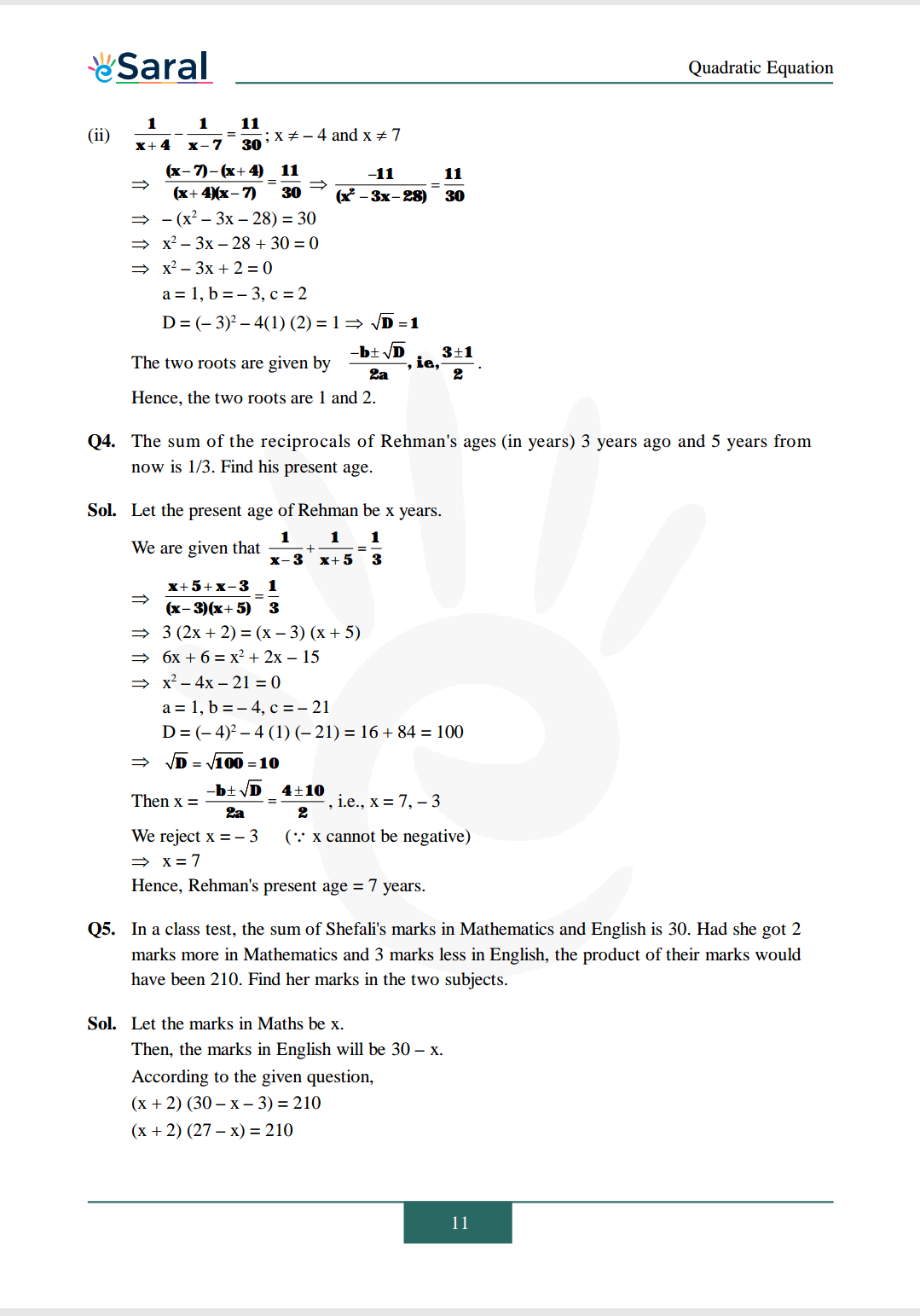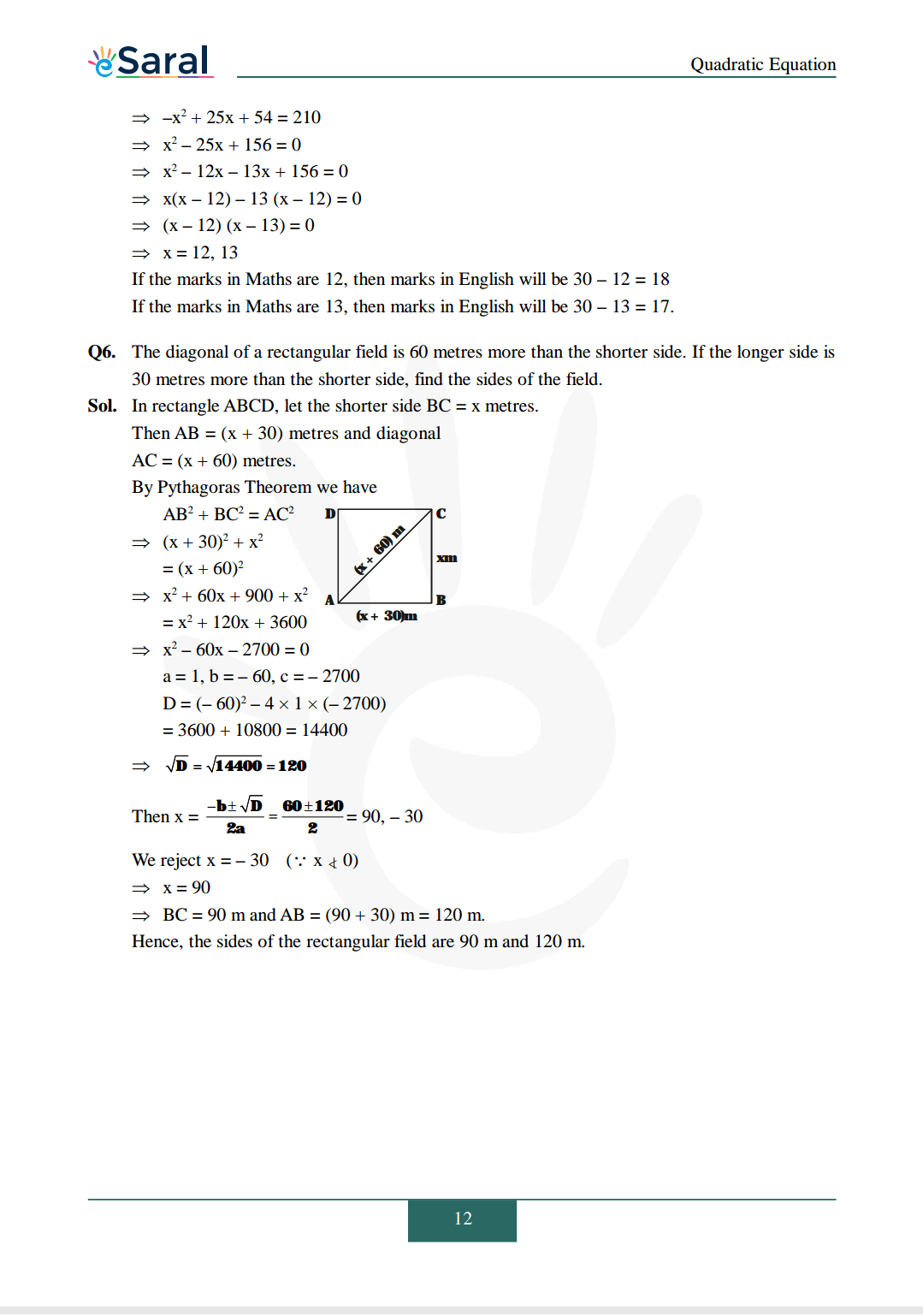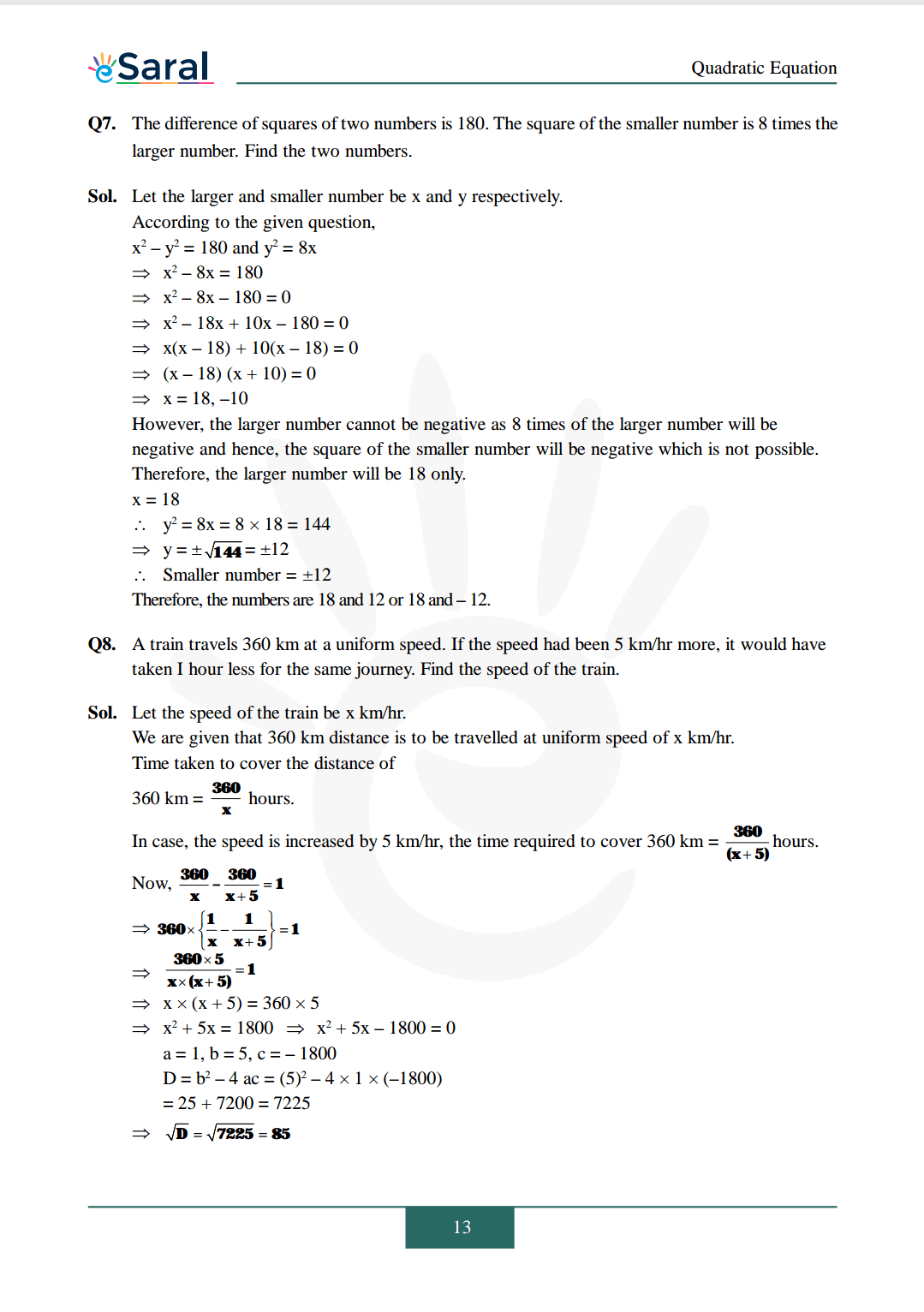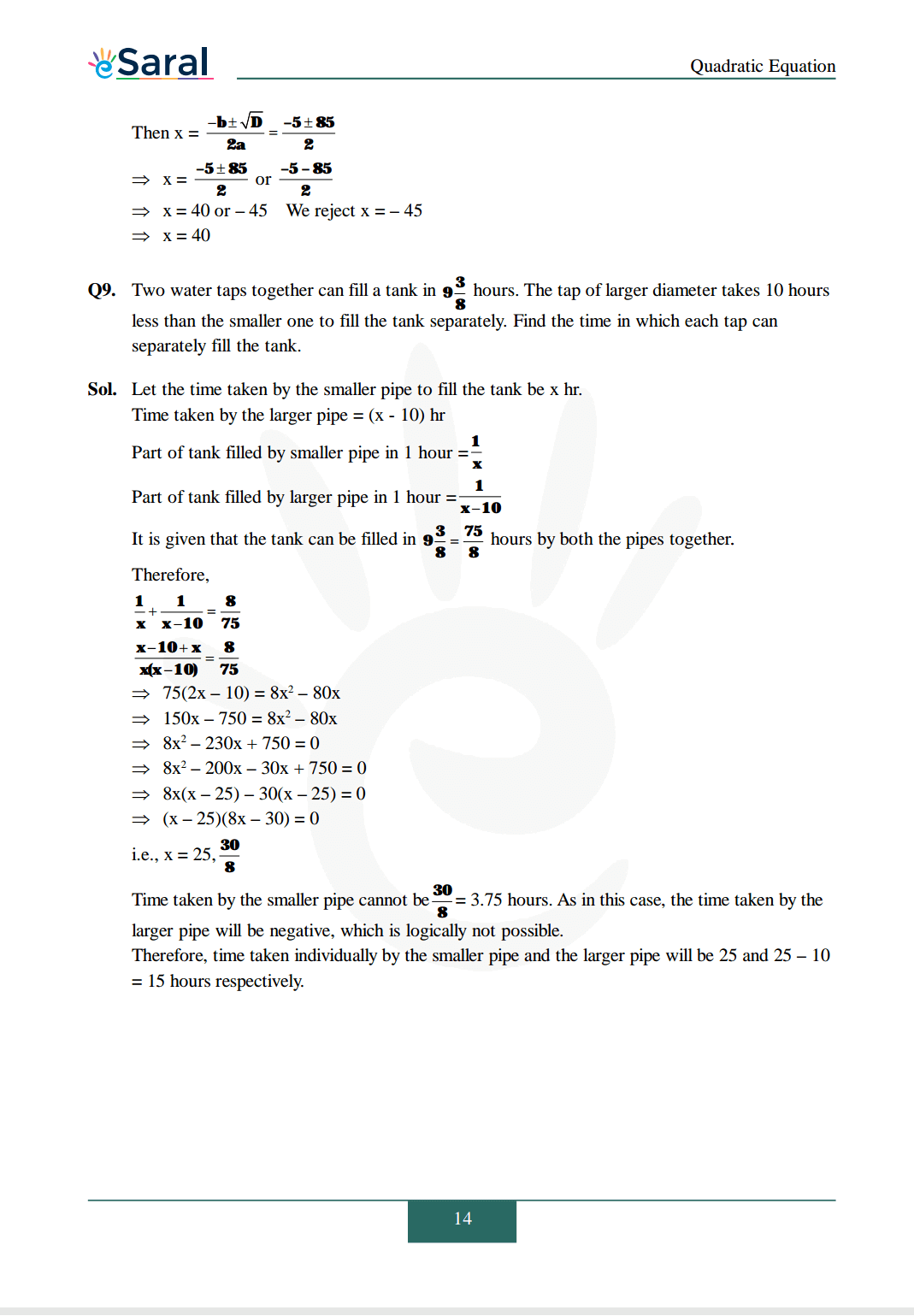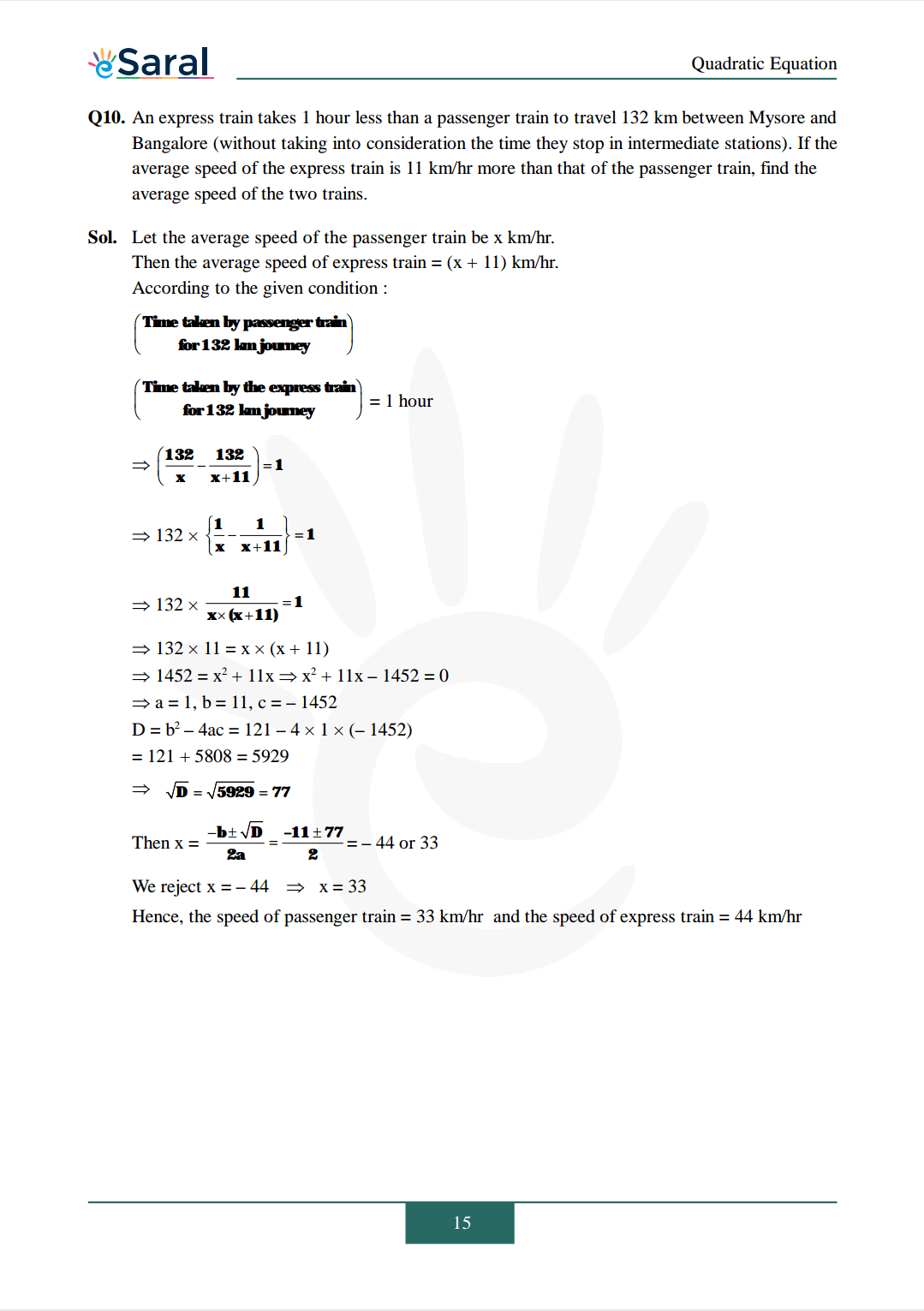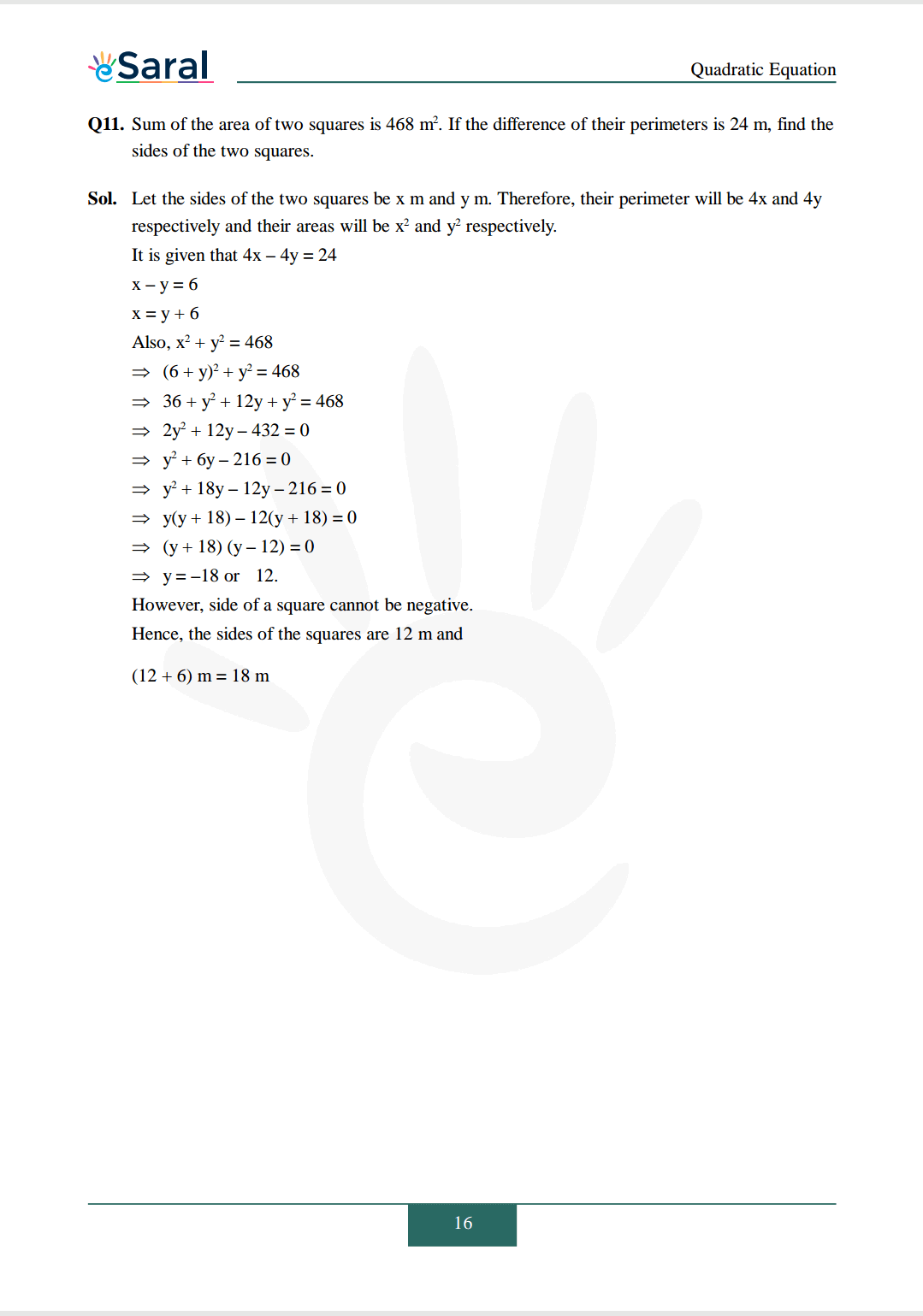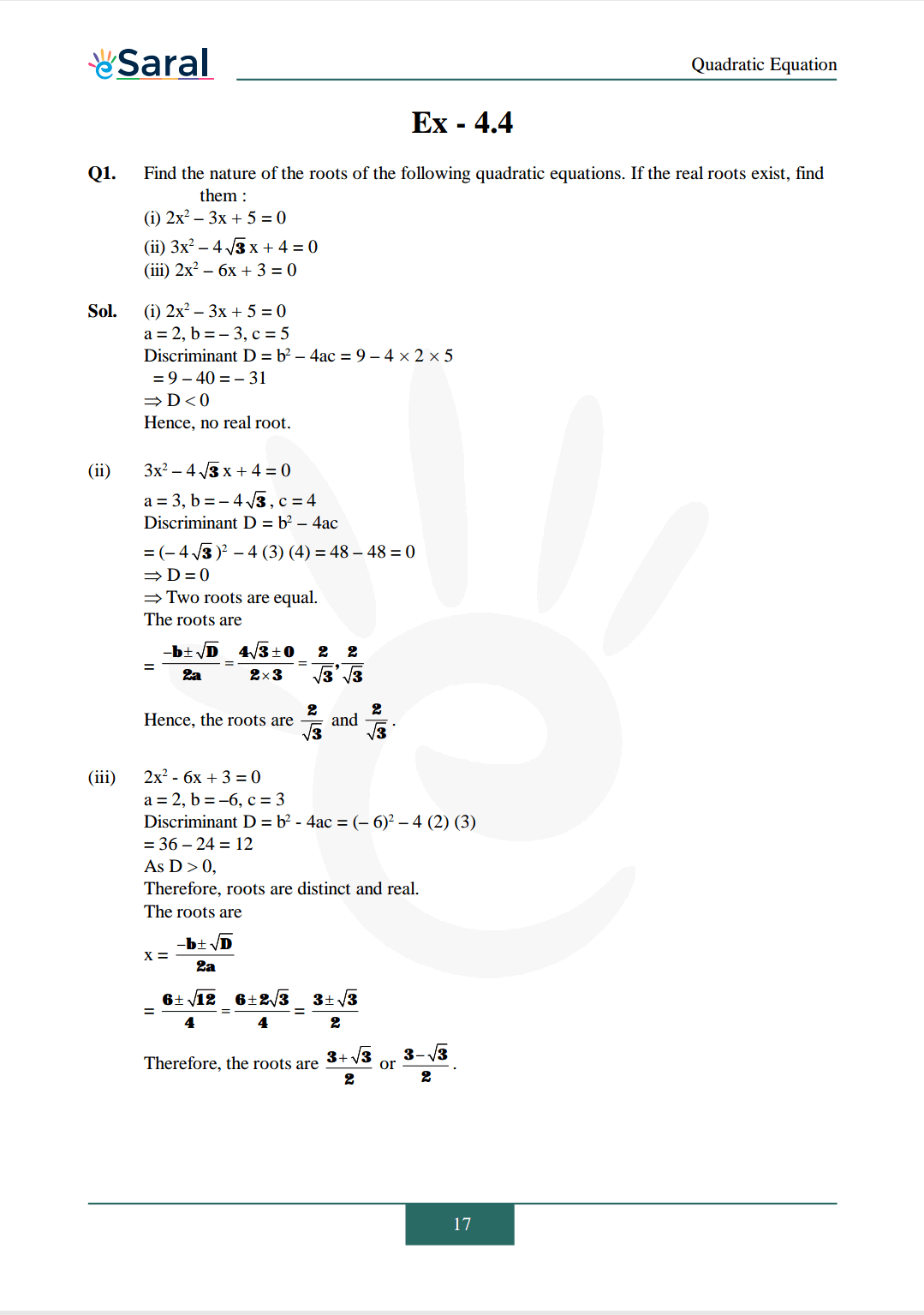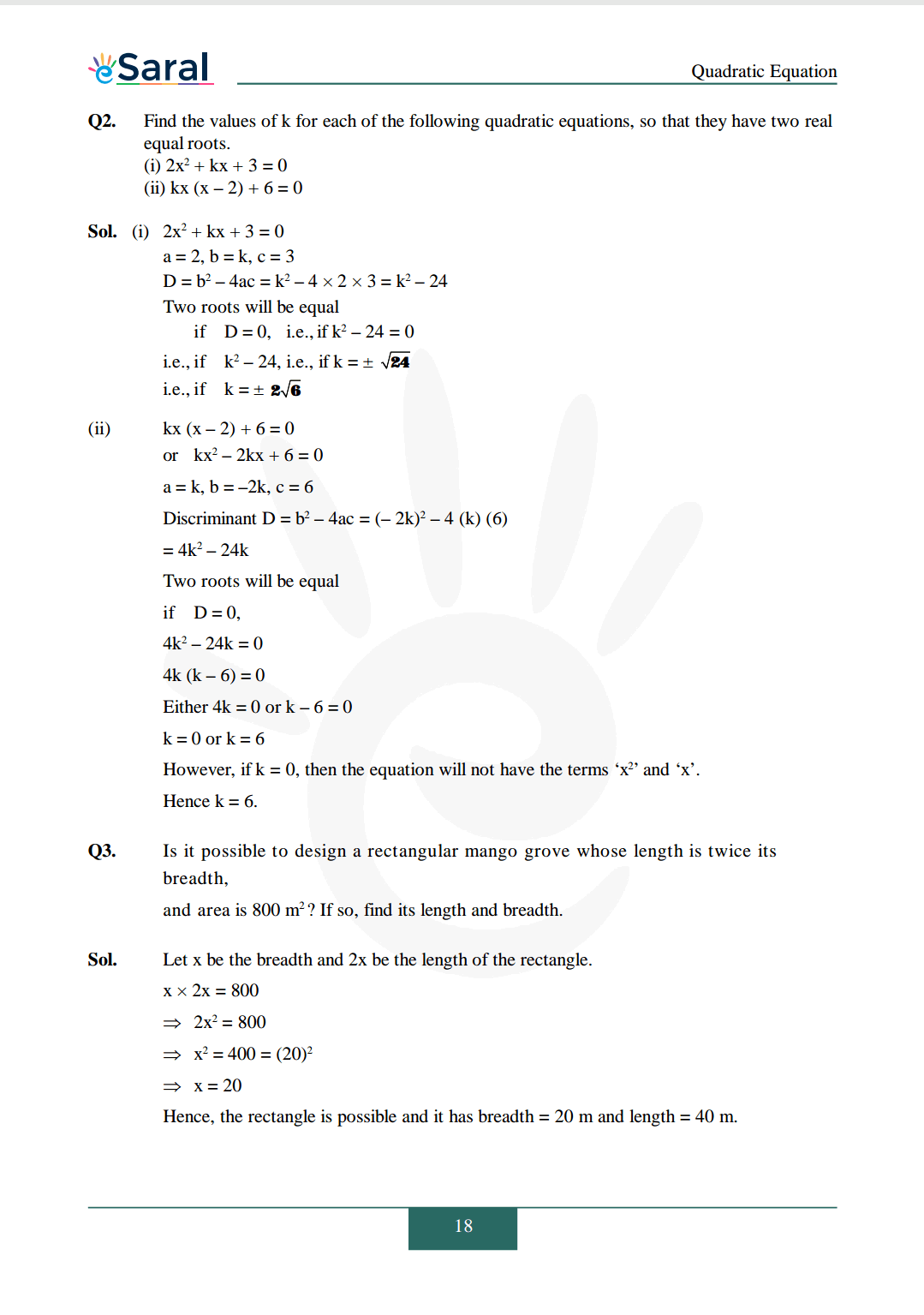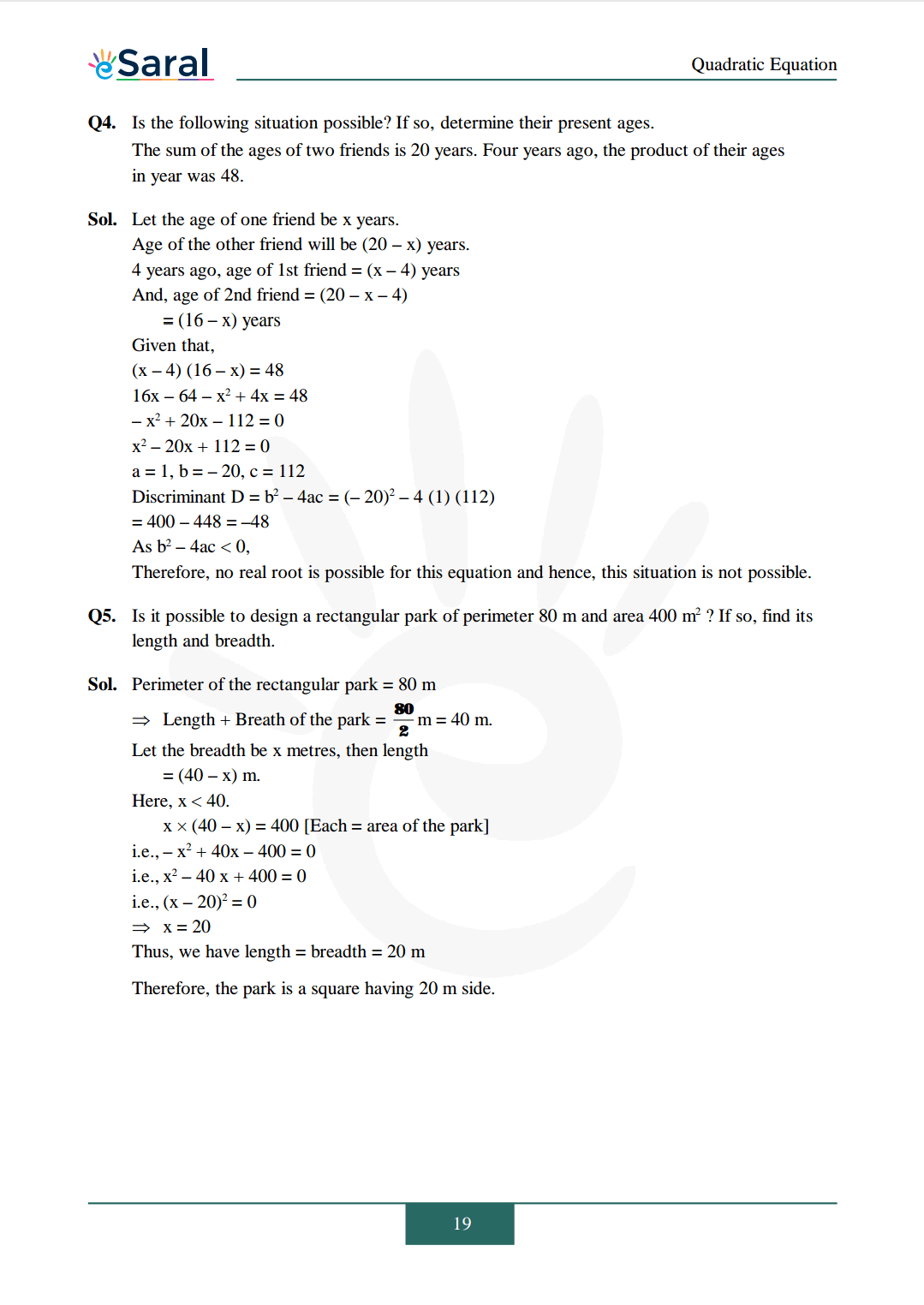Class 10 Maths Revision Short Notes.

If you have any Confusion related to NCERT Solutions for Class 10 Maths chapter 4 then feel free to ask in the comments section down below.

To watch Free Learning Videos on Class 10 by Kota’s top Faculties Install the eSaral App

## Q1.Is eSaral providing answers for NCERT Solutions quadratic equations class 10 Chapter 4?

A1.Yes, you can avail of the PDFs of NCERT Solutions for Class 10 Maths Quadratic Equations.Also, they provide solutions for Classes 1 to 12 NCERT Textbooks in free PDFs. Who wish to score high in CBSE board exams are advised to solve the NCERT Textbook

## Q2.Mention the important concepts you learn in NCERT Solutions quadratic equations class 10 Chapter 4?

A2.The concepts presented in NCERT Solutions for Class 10 Maths Chapter 4 Quadratic Equations are the meaning and definition of quadratic equations, finding the roots of quadratic equations by factorisation, finding the roots of quadratic equations by completing squares and the nature of the roots

## Q3.How many exercises are there in NCERT Solutions quadratic equations class 10 Chapter 4?

A3.The fourth chapter of NCERT Solutions for Class 10 Maths has 4 exercises. The first exercise deals with the topic of determining the quadratic equations, the second exercise has questions about finding the roots of quadratic equations by factorisation, the third exercise deals with finding the roots of quadratic equations by completing squares, and the last exercise has the question based on the nature of the roots. By solving these exercises, students are able to answer all the questions based on quadratic equations.